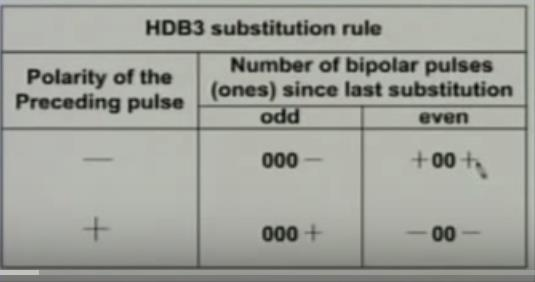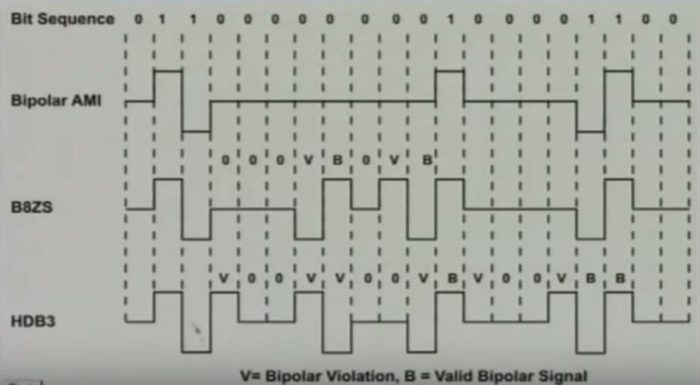# Line Coding Schemes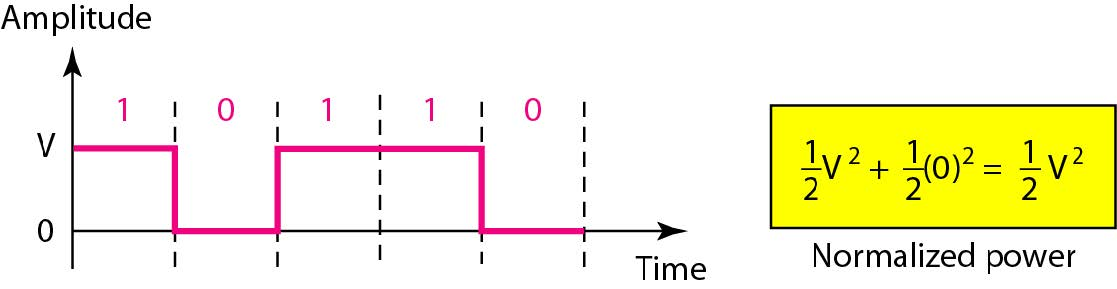September 18, 2016

Categorised in:

Line Coding

• Unipolar
• Only two voltage levels are used. (1 means sending something, 0 means not sending)
• All signal levels are on one side of the time axis – either above or below
• NRZ – Non Return to Zero scheme is an example of this code. The signal level does not return to zero during a symbol transmission.
• Unipolar NRZ Scheme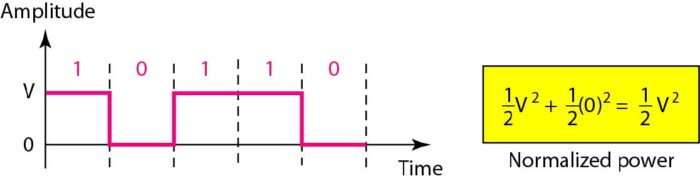• Polar
• The voltages are on the both sides of the time axis. Ex: Polar NRZ
• Polar alternatives : NRZ, RZ, Manchester, Differential Manchester
•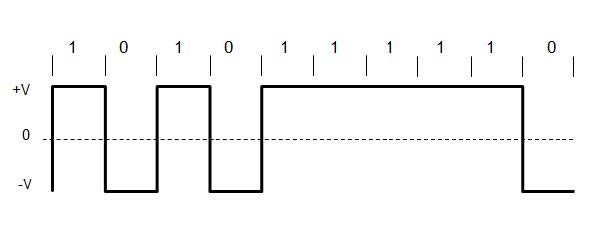• Polar NRZ scheme can be implemented with two voltages. E.g. +V for 1 and -V for 0.
• There are two versions:
• NZR – Level (NRZ-L) – 1 for low level and 0 for high level
• NRZ – Inversion (NRZ-I) – the inversion or the lack of inversion determines the value of the bit. (for each one in the bit sequence the signal level is inverted. )
•• Polar RZ ensures Synchronization, there must be a single transition in each bit.
• The Return to Zero (RZ) scheme uses three voltage values. +, 0, -.
•• Manchester coding consists of combining the NRZ-L and RZ schemes.
• In Manchester encoding there is a mid-bit transition serves as a clocking mechanism and also as data.
• Low to high represents a 1. High to low represents a 0.
• Differential Manchester coding consists of combining the NRZ-I and RZ schemes.
• Always a transition at the middle of the bit
• Presence of transition in the beginning of a bit represents 0.
•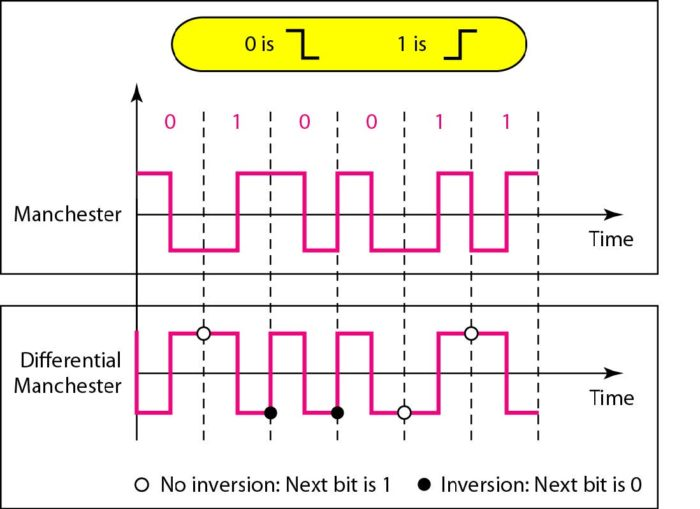• Bipolar Scheme
• Three voltage levels- positive, negative, and zero.
• The voltage level for one data element is at zero, while the voltage level for the other element alternates between positive and negative.
• Example : AMI and Pseudoternary
• Alternate mark inversion (AMI)
• A common bipolar encoding scheme is called bipolar alternate mark inversion (AMI). In alternate mark inversion, a neutral zero voltage represents binary O. Binary 1s are represented by alternating positive and negative voltages.
• Disadv : Synchronization fails when long sequence of 0.
•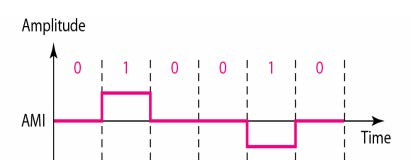• Pseudoternary
• A variation of AMI encoding is called Pseudoternary in which the 1 bit is encoded as a zero voltage and the 0 bit is encoded as alternating positive and negative voltages.
•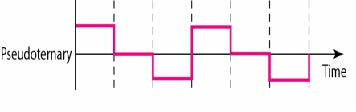Scrambling Schemes

• Extension of Bipolar AMI.
• Used in case of long distance applications.
• Goals :
• No DC Component
• No Long sequences of 0 level line signal.
• Minimum increase in bandwidth.
• Error detection capability.
• Example : B8ZS, HBD3

B8ZS (Bipolar with 8 zero substitution)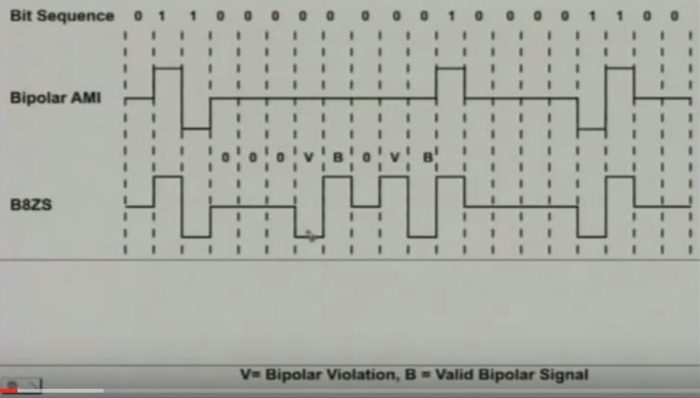HBD3(High Density Bipolar- 3 Zero’s